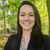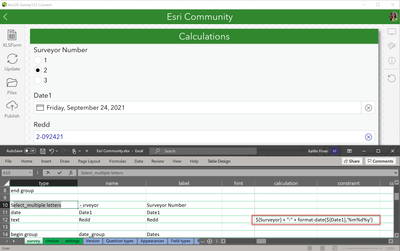Help labeling

91
1
09-21-2021 11:01 AMNew Contributor

I have a survey wherein a user selects their personal "Surveyor ID" from a select_one list of possible surveyor ID's (e.g. 13 - surveyor name) as well as the survey date.  Further down the form I have a text question wherein the surveyor can record the presence of a fish nest (i.e. a redd).  This is done by assigning a number to the redd.  Our typical protocol for assigning numbers is to use your personal Surveyor ID plus a six digit date and then a two digit number.  For example, if I was conducting a survey today and I observed a redd I would assign the first redd I observe with the following value: 13-092121-01.  The second redd would be: 13-092121-02.  My question is, is there a way to populate the "Redd number" question with the default answer of surveyor_id - survey_date - ## (i.e 13-092121-##), where the surveyor would only be inputing the last two digits?

1 Solution

Accepted SolutionsbyEsri Contributor

Hi Matt, this is certainly possible! You would add the survey and date field question, and then set up a calculation expression for the redd field that you want to autopopulate a combination of the survey number and date. Within the calculation field for that question, you can reference previous answer and reformat the data.

Here is a blog about formatting dates

Here is a screenshot of how you can set it upbyEsri Contributor

Hi Matt, this is certainly possible! You would add the survey and date field question, and then set up a calculation expression for the redd field that you want to autopopulate a combination of the survey number and date. Within the calculation field for that question, you can reference previous answer and reformat the data.

Here is a blog about formatting dates

Here is a screenshot of how you can set it up